SBOSA16 October   2020

PRODUCTION DATA

1. Features
2. Applications
3. Description
4. Revision History
5. Pin Configuration and Functions
6. Specifications
7. Detailed Description
1. 7.1 Overview
2. 7.2 Functional Block Diagram
3. 7.3 Feature Description
4. 7.4 Device Functional Modes
8. Application and Implementation
1. 8.1 Application Information
2. 8.2 Typical Applications
1. 8.2.1 High DAC Gain Stage for Semiconductor Test Equipment
2. 8.2.2 Improved Howland Current Pump for Bioimpedance Measurements in Multiparameter Patient Monitors
9. Power Supply Recommendations
10. 10Layout
1. 10.1 Layout Guidelines
2. 10.2 Layout Example
11. 11Device and Documentation Support
1. 11.1 Device Support
1. 11.1.1 Development Support
2. 11.2 Documentation Support
3. 11.3 Support Resources
5. 11.5 Electrostatic Discharge Caution
6. 11.6 Glossary
12. 12Mechanical, Packaging, and Orderable Information

• DDA|8
• DDA|8

## 6.6 Typical Characteristics

at TA = 25°C, VS = ±75 V, and RL = 10 kΩ connected to GND, output enabled (unless otherwise noted)

Table 6-1 Table of Graphs
DESCRIPTION FIGURE
Offset Voltage Distribution at 25°C Figure 6-1
Offset Voltage Distribution at 85°C Figure 6-2
Offset Voltage Distribution at -40°C Figure 6-3
Offset Voltage Drift Distribution from -40°C to +85°C Figure 6-4
Offset Voltage vs Temperature Figure 6-5
Offset Voltage Warmup Figure 6-6
Offset Voltage vs Common-Mode Voltage (Low Vcm) Figure 6-7
Offset Voltage vs Common-Mode Voltage (High Vcm) Figure 6-8
Offset Voltage vs Power Supply (Low Supply) Figure 6-9
Offset Voltage vs Power Supply (High Supply) Figure 6-10
Offset Voltage vs Output Voltage (Low Output) Figure 6-11
Offset Voltage vs Output Voltage (High Output) Figure 6-12
CMRR vs Temperature Figure 6-13
CMRR vs Frequency Figure 6-14
PSRR vs Temperature Figure 6-15
PSRR vs Frequency Figure 6-16
EMIRR vs Frequency Figure 6-17
No Phase Reversal Figure 6-18
Input Bias Current Production Distribution at 25℃ Figure 6-19
IB vs Temperature Figure 6-20
IB vs Common-Mode Voltage Figure 6-21
Enable Response Figure 6-22
Current Limit Response Figure 6-23
Open-Loop Gain vs Temperature Figure 6-24
Open-Loop Gain vs Output Voltage Figure 6-25
Open-Loop Gain and Phase vs Frequency Figure 6-26
Open-Loop Output Impedance vs Frequency Figure 6-27
Closed-Loop Gain vs Frequency Figure 6-28
Maximum Output Voltage vs Frequency Figure 6-29
Positive Output Voltage vs Output Current Figure 6-30
Negative Output Voltage vs Output Current Figure 6-31
Short-Circuit Current vs Temperature Figure 6-32
Settling Time Figure 6-35
Phase Margin vs Capacitive Load Figure 6-36
Small-Signal Overshoot vs Capacitive Load (G = –1) Figure 6-37
Small-Signal Overshoot vs Capacitive Load (G = +1) Figure 6-38
Small-Signal Step Response (G = –1) Figure 6-39
Small-Signal Step Response (G = +1) Figure 6-40
Large-Signal Step Response (G = –1) Figure 6-41
Large-Signal Step Response (G = +1) Figure 6-42
Slew Rate vs Output Step Size Figure 6-43
Slew Rate vs Supply Voltage (Inverting) Figure 6-44
Slew Rate vs Supply Voltage (Noninverting) Figure 6-45
THD+N Ratio vs Frequency (G = 10) Figure 6-46
THD+N Ratio vs Frequency (G = 20) Figure 6-47
THD+N Ratio vs Output Amplitude (G = 10) Figure 6-48
THD+N Ratio vs Output Amplitude (G = 20) Figure 6-49
0.1-Hz to 10-Hz Noise Figure 6-50
Input Voltage Noise Spectral Density Figure 6-51
Current Noise Density Figure 6-52
Quiescent Current Production Distribution at 25℃ Figure 6-53
Quiescent Current vs Supply Voltage Figure 6-54
Quiescent Current vs Temperature Figure 6-55
Status Flag Voltage vs Temperature Figure 6-56
Quiescent Current vs Enable Voltage Figure 6-57
Enable Current vs Enable Voltage Figure 6-58
Status Flag Current vs Voltage Figure 6-59Figure 6-1 Offset Voltage Distribution at 25°CFigure 6-3 Offset Voltage Distribution at –40°CFigure 6-5 Offset Voltage vs TemperatureFigure 6-7 Offset Voltage vs Common-Mode Voltage (Low VCM)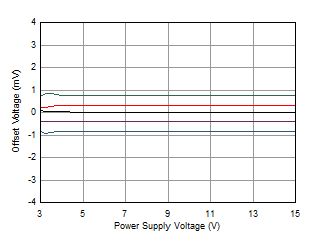Figure 6-9 Offset Voltage vs Power Supply
(Low Supply)Figure 6-11 Offset Voltage vs Output Voltage
(Low Output)Figure 6-13 CMRR vs TemperatureFigure 6-15 PSRR vs Temperature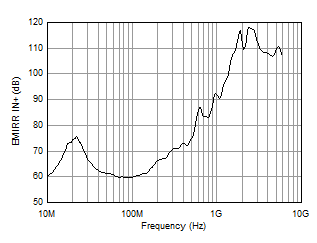Figure 6-17 EMIRR vs FrequencyFigure 6-19 Input Bias Current Production Distribution at 25℃Figure 6-21 IB vs Common-Mode Voltage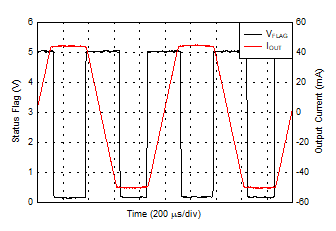Figure 6-23 Current Limit ResponseFigure 6-25 Open-Loop Gain vs Output VoltageFigure 6-27 Open-Loop Output Impedance vs FrequencyFigure 6-29 Maximum Output Voltage vs FrequencyFigure 6-31 Negative Output Voltage
vs Output CurrentFigure 6-35 Settling Time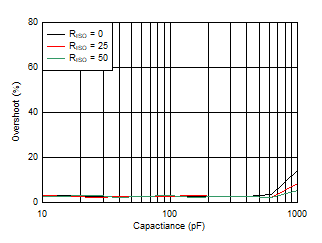G = –1
Figure 6-37 Small-Signal Overshoot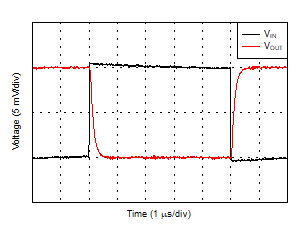G = –1
Figure 6-39 Small-Signal Step ResponseG = –1
Figure 6-41 Large-Signal Step ResponseFigure 6-43 Slew Rate vs Output Step SizeFigure 6-45 Slew Rate vs Supply Voltage (Noninverting)G = 20
Figure 6-47 THD+N Ratio vs FrequencyG = 20
Figure 6-49 THD+N Ratio vs Output AmplitudeFigure 6-51 Input Voltage Noise Spectral DensityFigure 6-53 Quiescent Current Production Distribution at 25℃Figure 6-55 Quiescent Current vs TemperatureFigure 6-57 Quiescent Current vs Enable VoltageFigure 6-59 Status Flag Current vs VoltageFigure 6-2 Offset Voltage Distribution at 85°CFigure 6-4 Offset Voltage Drift Distribution
From –40°C to +85°CFigure 6-6 Offset Voltage WarmupFigure 6-8 Offset Voltage vs Common-Mode Voltage (High VCM)Figure 6-10 Offset Voltage vs Power Supply
(High Supply)Figure 6-12 Offset Voltage vs Output Voltage
(High Output)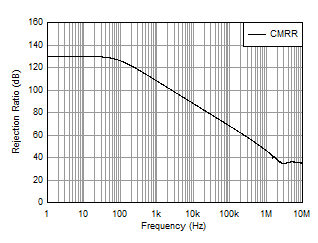Figure 6-14 CMRR vs FrequencyFigure 6-16 PSRR vs FrequencyFigure 6-18 No Phase ReversalFigure 6-20 IB vs TemperatureFigure 6-22 Enable ResponseFigure 6-24 Open-Loop Gain vs Temperature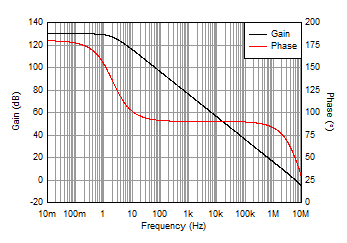Figure 6-26 Open-Loop Gain and Phase vs Frequency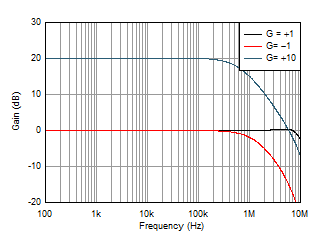Figure 6-28 Closed-Loop Gain vs FrequencyFigure 6-30 Positive Output Voltage
vs Output CurrentFigure 6-32 Short-Circuit Current vs Temperature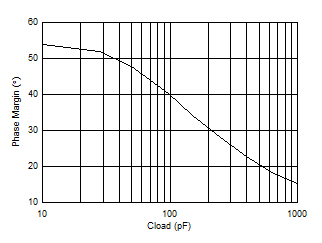Figure 6-36 Phase Margin vs Capacitive Load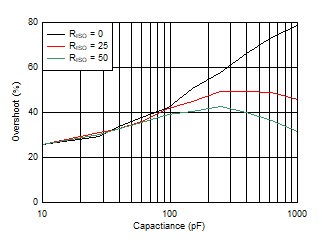G = +1
Figure 6-38 Small-Signal Overshoot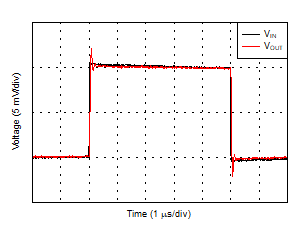G = +1
Figure 6-40 Small-Signal Step Response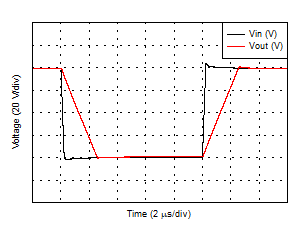G = +1
Figure 6-42 Large-Signal Step ResponseFigure 6-44 Slew Rate vs Supply Voltage (Inverting)G = 10
Figure 6-46 THD+N Ratio vs FrequencyG = 10
Figure 6-48 THD+N Ratio vs Output Amplitude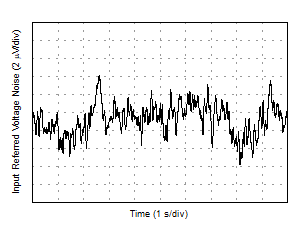Figure 6-50 0.1-Hz to 10-Hz NoiseFigure 6-52 Current Noise DensityFigure 6-54 Quiescent Current vs Supply VoltageFigure 6-56 Status Flag Voltage vs TemperatureFigure 6-58 Enable Current vs Enable Voltage